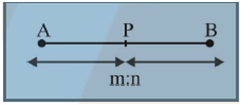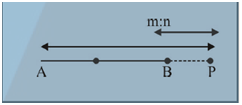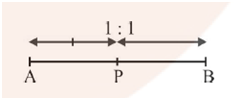#### If (2, 3, 5) is one end of a diameter of the spherethen the coordinates of the other end of the diameter are Option 1) (4, 3, 5) Option 2) (4, 3, –3) Option 3) (4, 9, –3) Option 4) (4, –3, 3)As we learnt in

Section Formula -

1)    Internal Division2)    External Division3)    Mid Point Formula- whereinCoordinate of centre of sphere= (3,6,1)

Let other end be (x,y,z)Option 1)

(4, 3, 5)

Incorrect Option

Option 2)

(4, 3, –3)

Incorrect Option

Option 3)

(4, 9, –3)

Correct Option

Option 4)

(4, –3, 3)

Incorrect Option

#### Plabita